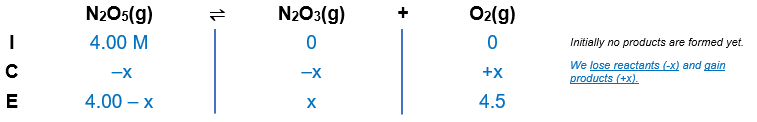# Problem: When N2O5(g) is heated it dissociates into N2O3(g) and O2(g) according to the following reaction:N2O5(g) ⇌ N2O3(g) + O2(g); Kc = 7.75 at a given temperatureThe N2O3(g) dissociates to give N2O(g) and O2(g) according the following reaction:N2O3(g) ⇌ N2O(g) + O2(g); Kc = 4.00 at the same temperatureWhen 4.00 mol of N2O5(g) is heated in a 1.00-L reaction vessel to this temperature, the concentration of O2(g) at equilibrium is 4.50 mol/L. Find the concentration of N2O3 in the equilibrium system.

###### FREE Expert Solution

$\overline{)\mathbf{Molarity}\mathbf{\text{(M)}}\mathbf{=}\frac{\mathbf{mol}}{\mathbf{L}}}$

N2O5(g) ⇌ N2O3(g) + O2(g);          Kc = 7.75

[N2O5] = 4.00 M${\mathbf{K}}_{\mathbf{c}}\mathbf{=}\frac{\mathbf{products}}{\mathbf{reactants}}\phantom{\rule{0ex}{0ex}}{\mathbf{K}}_{\mathbf{c}}\mathbf{=}\frac{\left[{N}_{2}{O}_{3}\right]\left[{O}_{2}\right]}{\left[{N}_{2}{O}_{5}\right]}\phantom{\rule{0ex}{0ex}}\mathbf{7}\mathbf{.}\mathbf{75}\mathbf{=}\frac{\left(x\right)\left(4.5\right)}{\left(4.00-x\right)}\phantom{\rule{0ex}{0ex}}\mathbf{7}\mathbf{.}\mathbf{75}\left(4.00-x\right)\mathbf{=}\mathbf{4}\mathbf{.}\mathbf{5}\mathbf{x}\phantom{\rule{0ex}{0ex}}\mathbf{31}\mathbf{-}\mathbf{7}\mathbf{.}\mathbf{75}\mathbf{x}\mathbf{=}\mathbf{4}\mathbf{.}\mathbf{5}\mathbf{x}\phantom{\rule{0ex}{0ex}}\mathbf{31}\mathbf{=}\mathbf{7}\mathbf{.}\mathbf{75}\mathbf{x}\mathbf{+}\mathbf{4}\mathbf{.}\mathbf{5}\mathbf{x}\phantom{\rule{0ex}{0ex}}\frac{\mathbf{31}}{\mathbf{12}\mathbf{.}\mathbf{25}}\mathbf{=}\frac{\overline{)\mathbf{12}\mathbf{.}\mathbf{25}}\mathbf{x}}{\overline{)\mathbf{12}\mathbf{.}\mathbf{25}}}$

x = 2.5306

x = [N2O3]

[N2O3] = 2.5306 M

N2O3(g) ⇌ N2O(g) + O2(g);          Kc = 4.00

82% (373 ratings)###### Problem Details

When N2O5(g) is heated it dissociates into N2O3(g) and O2(g) according to the following reaction:

N2O5(g) ⇌ N2O3(g) + O2(g); Kc = 7.75 at a given temperature

The N2O3(g) dissociates to give N2O(g) and O2(g) according the following reaction:

N2O3(g) ⇌ N2O(g) + O2(g); Kc = 4.00 at the same temperature

When 4.00 mol of N2O5(g) is heated in a 1.00-L reaction vessel to this temperature, the concentration of O2(g) at equilibrium is 4.50 mol/L. Find the concentration of N2O3 in the equilibrium system.